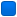## what does d E actually mean in the output?

Question on input files/tags, interpreting output, etc.

Please check whether the answer to your question is given in the VASP online manual or has been discussed in this forum previously!

Moderators: Global Moderator, Moderator

Message
Author
guoxingmiao
NewbiePosts: 4
Joined: Mon Nov 18, 2019 1:56 pm

### what does d E actually mean in the output?

I had assumed it means the change of energy between steps (as in the VASP tuitorial: "dE is the change in the total energy between the current and the last step"), until recently I noticed it is nowhere close to such value. For example, I have a hard time converging an ion relaxation: the F and E0 virtually stopped changing (some <10^-4 variaztion) while d E still reports 10^-2 each step. Not surprisingly, it does not converge as I set EDIFF=0.001. For example, as below, the change between the last two E0 is 0.000005, while d E reports -0.018 so it does not converge. Is this normal?

34 F= -.76747776E+02 E0= -.76739597E+02 d E =-.180560E-01
curvature: -0.00 expect dE=-0.572E-05 dE for cont linesearch -0.322E-06
ZBRENT: interpolating
opt : 7.7535 next Energy= -76.748184 (dE=-0.185E-01)
bond charge predicted
N E dE d eps ncg rms rms(c)
DAV: 1 -0.767478208820E+02 -0.67046E-03 -0.43908E-03 5256 0.551E-01 0.464E-02
DAV: 2 -0.767494366242E+02 -0.16157E-02 -0.56860E-03 3384 0.117E+00 0.864E-02
DAV: 3 -0.767477339355E+02 0.17027E-02 -0.37551E-03 3408 0.991E-01 0.167E-02
DAV: 4 -0.767478741776E+02 -0.14024E-03 -0.13694E-03 3216 0.963E-02
35 F= -.76747874E+02 E0= -.76739709E+02 d E =-.181539E-01
curvature: -0.04 expect dE=-0.915E-04 dE for cont linesearch -0.244E-05
ZBRENT: bisectioning
opt : 7.7287 next Energy= -76.747887 (dE=-0.182E-01)
bond charge predicted
N E dE d eps ncg rms rms(c)
DAV: 1 -0.767478675938E+02 -0.13366E-03 -0.84346E-04 3192 0.509E-02 0.174E-02
DAV: 2 -0.767478686959E+02 -0.11021E-05 -0.85529E-05 3072 0.309E-02
36 F= -.76747869E+02 E0= -.76739718E+02 d E =-.181484E-01
curvature: -0.21 expect dE=-0.671E-03 dE for cont linesearch -0.277E-03
ZBRENT: interpolating
opt : 7.7491 next Energy= -76.747875 (dE=-0.182E-01)
bond charge predicted
N E dE d eps ncg rms rms(c)
DAV: 1 -0.767478814259E+02 -0.13832E-04 -0.35047E-04 3192 0.113E-01 0.188E-02
DAV: 2 -0.767479990997E+02 -0.11767E-03 -0.51165E-04 3216 0.344E-01
37 F= -.76747999E+02 E0= -.76739879E+02 d E =-.182788E-01
curvature: -0.00 expect dE=-0.223E-05 dE for cont linesearch -0.501E-06
ZBRENT: bisectioning
opt : 7.7389 next Energy= -76.748027 (dE=-0.183E-01)
bond charge predicted
N E dE d eps ncg rms rms(c)
DAV: 1 -0.767478884676E+02 -0.70417E-05 -0.34511E-04 3192 0.289E-01 0.607E-03
DAV: 2 -0.767479431266E+02 -0.54659E-04 -0.19609E-04 3096 0.208E-01
38 F= -.76747943E+02 E0= -.76739809E+02 d E =-.182228E-01
curvature: -0.04 expect dE=-0.996E-04 dE for cont linesearch -0.204E-04
ZBRENT: interpolating
opt : 7.7436 next Energy= -76.747946 (dE=-0.182E-01)
bond charge predicted
N E dE d eps ncg rms rms(c)
DAV: 1 -0.767478865395E+02 0.19281E-05 -0.11446E-04 3096 0.167E-01 0.128E-03
DAV: 2 -0.767478924109E+02 -0.58715E-05 -0.54410E-05 3072 0.133E-02
39 F= -.76747892E+02 E0= -.76739733E+02 d E =-.181721E-01
curvature: -0.01 expect dE=-0.156E-04 dE for cont linesearch -0.344E-05
ZBRENT: bisectioning
opt : 7.7463 next Energy= -76.747896 (dE=-0.182E-01)
bond charge predicted
N E dE d eps ncg rms rms(c)
DAV: 1 -0.767478967494E+02 -0.10210E-04 -0.19734E-05 2640 0.103E-02 0.252E-03
DAV: 2 -0.767478972856E+02 -0.53619E-06 -0.31254E-06 2904 0.442E-03
40 F= -.76747897E+02 E0= -.76739738E+02 d E =-.181770E-01
curvature: -0.01 expect dE=-0.176E-04 dE for cont linesearch -0.408E-05
ZBRENT: bisectioning
opt : 7.7477 next Energy= -76.747899 (dE=-0.182E-01)

marie-therese.huebsch
Global ModeratorPosts: 51
Joined: Tue Jan 19, 2021 12:01 am

### Re: what does d E actually mean in the output?

Dear guoxingmiao,

Sorry for the late response!

dE always refers to some energy difference, but as there are many selfconsistency loops and minimization algorithms, e.g., the ionic relaxation, electronic relaxation, line minimization, and the diagonalization of the Kohn–Sham Hamiltonian, it might not be obvious which energy difference it refers to. A more detailed summary of each relaxation step can be found in the OUTCAR file!

Also, note that the electronic convergence criterion is set by the EDIFF tag, and the ionic convergence criterion is controlled by EDIFFG! Please read their documentation on the VASP Wiki.

Please try to understand your calculation using the information written in the OUTCAR file. If you still have questions, you can upload your INCAR, KPOINTS, POTCAR, and POSCAR file, as well as the corresponding OUTCAR file here and I will be help you interpret the information.

Best regards,
Marie-Therese